Series v1.12

Series expansions for the TI-89/92+

Author: Bhuvanesh Bhatt (bbhatt1@towson.edu)

Last updated: October 3, 2001

nSeries(f(x), x, x0, n [,r])
calculates a numerical series expansion of f(x) about the point x = x0 to order n.

Here are some examples (in Float-4 precision):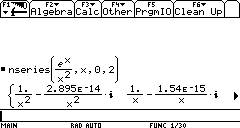The function 1/ln(x) has a singularity at x = 0, so its negative-power series never terminates: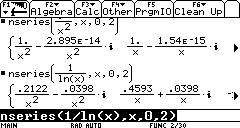This calculation doesn’t work, because of infinities that crop up in intermediate steps: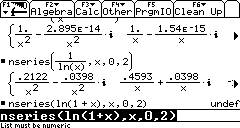However, in some cases you can get a result by varying the radius used: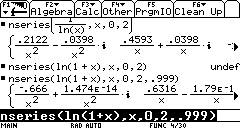Here is another example where the series expansion has an imaginary part: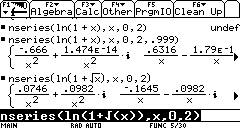Currently, there can be no symbolic variables except the independent variable: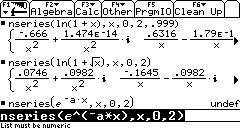Here is the Laurent series of e(-1/x^2) about x = 0: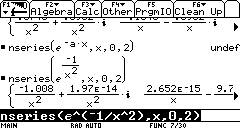The function 1/tan(x) has a simple pole, because the only nonzero negative-power
coefficient is that of 1/x:Here, the radius is too large, and so we get an incorrect value for the residue (-1.917):Reducing the radius gives a more accurate value (the exact value is -p2/3):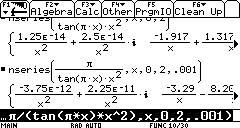What’s New:

§         The “List must be numeric” problem when evaluating nseries(ln(1+sqrt(x)),x,0,2) has been fixed.

§         The “List must be numeric” problem when using a variable other than x for the expansion has been fixed.

§         The bug with incorrect imaginary part in results has been fixed.

Notes:

§         nSeries() returns a list, because the AMS automatically combines the real and imaginary parts into the form realpart+imagpart·i. If you wish, you can use sum() to sum the list and get the “final” result.

§         There will often be small nonzero components in the result.

§         The default for the radius (r) argument in the nSeries() function is 1. You can change it, but take care that you don’t set it too small (cancellation errors can occur) or too large (artificial poles can start appearing).

Thanks to:

§         E.W., for inspiration J

§         Kevin Kofler, for his tip on using “volatile ESI” to refresh its value when called

§         My friends from the TI discussion groups

Future plans:

§         Implement rounding mechanism for small nonzero coefficients

§         Handle asymptotic expansions (numerical) directly

§         Symbolic series expansions (more general than the built-in taylor() function)

Disclaimer:

Although this program has been tested, it is still remotely possible that it may crash or hang. I am not responsible for any damage done to your calculator. To reset it, press:

[2nd][LOCK][ON] on the TI-92 Plus,
[2nd][LEFT][RIGHT][ON] on the TI-89

If this doesn't reset the calculator, take out a battery, press and hold [(-)][)] while you insert the battery. Of course, this does not mean your calculator will definitely crash or hang J In fact, you should be able to break a calculation by pressing [ON].